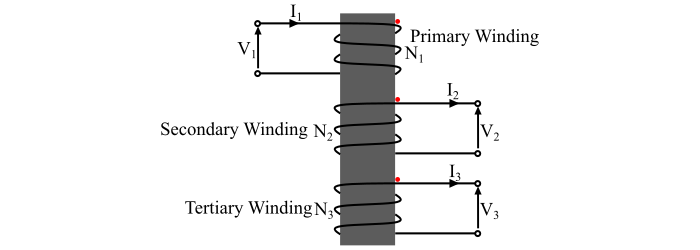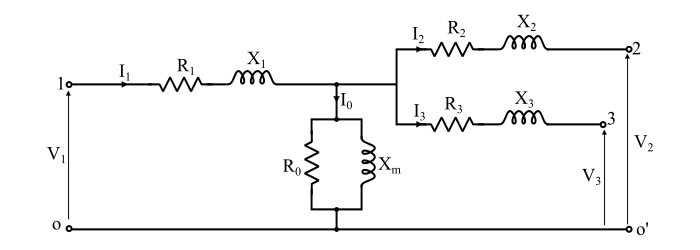# What is a Three Winding Transformer?

The transformers may also be constructed with a third winding in addition to the primary and secondary windings. This third winding is known as tertiary winding. The primary winding has the highest voltage rating, the tertiary winding has the lowest voltage rating and the secondary winding has the intermediate voltage rating. Also, the kVA ratings of the three windings of the 3-winding transformer are usually unequal. The figure shows the schematic diagram of a 3-winding transformer.The tertiary winding is always connected in delta. The primary advantage of the delta connected tertiary winding is that it suppresses any harmonic components that may be generated in the star connected primary and secondary windings of the transformer.

In case of unbalanced load currents in the secondary winding, which are reflected as unbalanced primary currents, an increased circulating current is reduced in the tertiary windings. This tends to restore the primary and secondary phase voltages to their normal magnitudes and phase angles. Therefore, the tertiary winding also reduces the secondary and primary imbalance and distributes the secondary load imbalance evenly among the primary phases.

The principle uses of the tertiary winding of the transformer are given as follows −

• The tertiary windings are used as auxiliary winding for supplying the substation uses (e.g., lighting, fan, pumps, etc.).

• Tertiary windings are used to interconnect three supply systems operating at different voltage levels.

• Tertiary windings can be used for measuring voltage of high voltage testing transformers.

• The tertiary windings may also be used for supplying a single load from two source, where the continuity of supply is important.

• Since the tertiary winding is connected in delta, thus it reduces the impedance offered to the zero-sequence currents to allow sufficient earth fault current for proper operation of protective devices.

• The synchronous condenser or static high voltage capacitors are connected across the delta connected output of the tertiary windings for reactive power injection into the power system for either power factor correction or voltage regulation or both.

The main advantages of a three winding transformer are greater efficiency and economical construction.

## Voltage Ratios and MMF Balance Equation

For an ideal three winding transformer, the voltage ratios are defined as follows −

$$\mathrm{\frac{𝑉_{2}}{𝑉_{1}}=\frac{𝑁_{2}}{𝑁_{1}}}$$

Where,

• V1 = Primary voltage,

• V2 = Secondary voltage,

• N1 = Number of turns in primary winding, and

• N2 = Number of turns in secondary winding.

Similarly, the voltage transformation ratio between primary and tertiary winding is

$$\mathrm{\frac{𝑉_{3}}{𝑉_{1}}=\frac{𝑁_{3}}{𝑁_{1}}}$$

Where,

• V3 is voltage across tertiary winding, and
• N3 is number of turns in tertiary winding.

Also, the total MMF of the primary winding is equal to the sum of MMF required by the secondary winding and MMF required by the tertiary winding, i.e.,

$$\mathrm{𝑁_{1}𝐼_{1} = 𝑁_{2}𝐼_{2} + 𝑁_{3}𝐼_{3}}$$

This expression is the MMF balance equation of a three winding transformer.

## Equivalent Circuit of Three Winding Transformer

The equivalent circuit of the 3-winding transformer is shown in the figure. The equivalent circuit of a 3-winding transformer can be represented by the 1-phase equivalent circuit, in which each winding of the transformer can be represented by its equivalent resistance and reactance.Here, the terminals 1, 2, 3 indicate primary, secondary and tertiary winding terminals respectively. The resistances R1, R2 and R3 are the resistances of primary, secondary and tertiary windings respectively. The reactances X1, X2 and X3 are the leakage reactances of the primary, secondary and tertiary windings respectively. If the no-load current is also considered, then the core loss resistance R0 and magnetising reactance Xm are also connected.